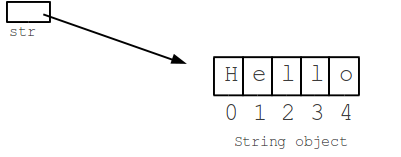CodingBat code practice

## Java String Introduction

Video

Strings are an incredibly common type of data in computers. This page introduces the basics of Java strings: chars, +, length(), and substring().

A Java string is a series of characters gathered together, like the word "Hello", or the phrase "practice makes perfect". Create a string in the code by writing its chars out between double quotes.

• String stores text -- a word, an email, a book
• All computer languages have strings, look similar
• "In double quotes"
• Sequence of characters ("char")
```String str = "Hello";
```

This picture shows the string object in memory, made up of the individual chars H e l l o. We'll see what the index numbers 0, 1, 2 .. mean later on.## String + Concatenation

Tthe + (plus) operator between strings puts them together to make a new, bigger string. The bigger string is just the chars of the first string put together with the chars of the second string.

```String a = "kit" + "ten";  // a is "kitten"
```

Strings are not just made of the letters a-z. Chars can be punctuation and other miscellelaneous chars. For example in the string "hi ", the 3rd char is a space. This all works with strings stored in variables too, like this:

```String fruit = "apple";
String stars = "***";
String a = fruit + stars;  //  a is "apple***"
```

CodingBat Practice> helloName

## String Length

The "length" of a string is just the number of chars in it. So "hi" is length 2 and "Hello" is length 5. The length() method on a string returns its length, like this:

```String a = "Hello";
int len = a.length();  // len is 5
```

## String Index Numbers

• Index numbers -- 0, 1, 2, ...
• Leftmost char is at index 0
• Last char is at index length-1The chars in a string are identified by "index" numbers. In "Hello" the leftmost char (H) is at index 0, the next char (e) is at index 1, and so on. The index of the last char is always one less than the length. In this case the length is 5 and 'o' is at index 4. Put another way, the chars in a string are at indexes 0, 1, 2, .. up through length-1. We'll use the index numbers to slice and dice strings with substring() in the next section.

## String Substring v1

• str.substring(start)
• Chars beginning at index start
• Through the end of the string
• Later: more complex 2-arg substring()The substring() method picks out a part of string using index numbers to identify the desired part. The simplest form, substring(int start) takes a start index number and returns a new string made of the chars starting at that index and running through the end of the string:

```String str = "Hello";
String a = str.substring(1);  // a is "ello" (i.e. starting at index 1)
String b = str.substring(2);  // b is "llo"
String c = str.substring(3);  // c is "lo"
```

Above `str.substring(1)` returns "ello", picking out the part of "Hello" which begins at index 1 (the "H" is at index 0, the "e" is at index 1).

CodingBat Practice> backAround

More problems to try

CodingBat.com code practice. Copyright 2012 Nick Parlante.